https://doi.org/10.5194/esurf-6-77-2018
https://doi.org/10.5194/esurf-6-77-2018

# Numerical modelling of landscape and sediment flux response to precipitation rate change

John J. Armitage, Alexander C. Whittaker, Mustapha Zakari, and Benjamin Campforts
Abstract

Laboratory-scale experiments of erosion have demonstrated that landscapes have a natural (or intrinsic) response time to a change in precipitation rate. In the last few decades there has been growth in the development of numerical models that attempt to capture landscape evolution over long timescales. However, there is still an uncertainty regarding the validity of the basic assumptions of mass transport that are made in deriving these models. In this contribution we therefore return to a principal assumption of sediment transport within the mass balance for surface processes; we explore the sensitivity of the classic end-member landscape evolution models and the sediment fluxes they produce to a change in precipitation rates. One end-member model takes the mathematical form of a kinetic wave equation and is known as the stream power model, in which sediment is assumed to be transported immediately out of the model domain. The second end-member model is the transport model and it takes the form of a diffusion equation, assuming that the sediment flux is a function of the water flux and slope. We find that both of these end-member models have a response time that has a proportionality to the precipitation rate that follows a negative power law. However, for the stream power model the exponent on the water flux term must be less than one, and for the transport model the exponent must be greater than one, in order to match the observed concavity of natural systems. This difference in exponent means that the transport model generally responds more rapidly to an increase in precipitation rates, on the order of 105 years for post-perturbation sediment fluxes to return to within 50 % of their initial values, for theoretical landscapes with a scale of 100×100 km. Additionally from the same starting conditions, the amplitude of the sediment flux perturbation in the transport model is greater, with much larger sensitivity to catchment size. An important finding is that both models respond more quickly to a wetting event than a drying event, and we argue that this asymmetry in response time has significant implications for depositional stratigraphies. Finally, we evaluate the extent to which these constraints on response times and sediment fluxes from simple models help us understand the geological record of landscape response to rapid environmental changes in the past, such as the Paleocene–Eocene thermal maximum (PETM). In the Spanish Pyrenees, for instance, a relatively rapid (10 to 50 kyr) duration of the deposition of gravel is observed for a climatic shift that is thought to be towards increased precipitation rates. We suggest that the rapid response observed is more easily explained through a diffusive transport model because (1) the model has a faster response time, which is consistent with the documented stratigraphic data, (2) there is a high-amplitude spike in sediment flux, and (3) the assumption of instantaneous transport is difficult to justify for the transport of large grain sizes as an alluvial bedload. Consequently, while these end-member models do not reproduce all the complexity of processes seen in real landscapes, we argue that variations in long-term erosional dynamics within source catchments can fundamentally control when, how, and where sedimentary archives can record past environmental change.

Share
Dates
1 Introduction

How river networks form and how landscapes erode remains a basic research question despite more than a century of experimentation and study. At a fundamental level, the root of the problem is a lack of an equation of motion for erosion derived from first principles (Dodds and Rothman2000). A range of heuristic erosion equations have, however, been proposed from stochastic models (Banavar et al.1997; Pastor-Satorras and Rothman1998) to deterministic models based on the St. Venant shallow water equations (Izumi and Parker1995; Smith2010; Smith and Bretherton1972), diffusive transport-limited conditions (Whipple and Tucker2002), or the stream power law (e.g. , among many others). These models, in various forms, have been explored to try to understand how landscape evolves and responds to tectono-environmental change. In general terms, numerical studies have found that landscapes typically recover from a shift in tectonic uplift after 105 to 106 years (reviewed in ). These apparently long response timescales to tectonic perturbations have been supported by field observations of landscapes upstream of active faults (Cowie et al.2008; Whittaker and Boulton2012; Whittaker et al.2007), although the precise appropriateness of any time-integrated erosion law to specific field sites is not always easy to establish. Sediment flux response times for the advective stream power law have been previously characterized by and , and for the transport model they have been studied by and , but not systematically or using 2-D models. Furthermore, to our knowledge no comparison between the transport models has been previously made.

The response of landscapes and sediment routing systems to a change in the magnitude or timescale of precipitation rates is expected to depend on the long-term erosion law implemented . Some numerical modelling studies based on treating erosion as a length-dependent diffusive problem suggest that landscape responses to a change in rainfall are also on the order of 105 to 106 years, similar to tectonic perturbations, although they produce diagnostically different stratigraphic signatures from the latter (Armitage et al.2011). However, other modelling contributions with different assumptions suggest that response times to a precipitation change may be more rapid , although field data sets remain equivocal (see , for a recent review). In laboratory studies, a series of experiments in which granular piles of a length scale of the order of centimetres are eroded due to surface water have demonstrated that a change in precipitation rate leads a period of adjustment of the landscape topography until a new steady state is achieved (Bonnet and Crave2003; Rohais et al.2011). These experiments use a mixture of granular silica of a mean diameter between 10 and 20 µm that is eroded by water released from a fine sprinkler system above. Given the complexity of these experiments, unfortunately there have been insufficient different precipitation rates studied to fully understand how the recovery timescale varies as a function of precipitation or other parameters.

It has been increasingly recognized over the last 2 decades that many basic geomorphic measures of catchments, such as the scaling between channel slopes and catchment drainage areas, are typically unable to distinguish the erosional processes behind their formation (Dodds and Rothman2000; Tinkler and Whol1998; Tucker and Whipple2002; Whipple2004). Erosion and transport can be described by equations that encompass both advective and diffusive processes (Smith and Bretherton1972) and at topographic steady state, it is very well established that fluvial erosion models based on either of these two end-members can produce very similar river longitudinal profiles (Tucker and Whipple2002; van der Beek and Bishop2003).

Non-uniqueness or equifinality is a common problem when comparing the morphology generated from landscape evolution models (Hancock et al.2016). Consequently, we aim to explore whether the sediment flux responses of fluvial systems to a precipitation perturbation may be diagnostically different for the two end-member deterministic models across a range of parameter space. This issue is pertinent because within sedimentary basins, a change in the erosional dynamics upstream could be recorded by changes in the total sediment volumes stored in sedimentary basins (Allen et al.2013; Michael et al.2014), in sediment delivery or sediment accumulation rates linked to landscape response times , and/or in the grain sizes deposited as a function of sediment flux output .

In this article we make a comparative study between the transport and stream power model to further explore the potential differences between these two end-member hypothetical landscape evolution models. We will focus on the transient period of adjustment to a perturbation in precipitation rates, and using end-member numerical models we attempt to evaluate how the response time varies as a function of the model forcing. To this end we aim to find the model parameters that generate similar landscape morphologies such that we can subsequently explore how the same end-member models respond to a change in precipitation rate. We believe that the results of this study have implications for understanding the responses of landscapes to past changes in climate and could potentially be compared with and tested against further laboratory experiments.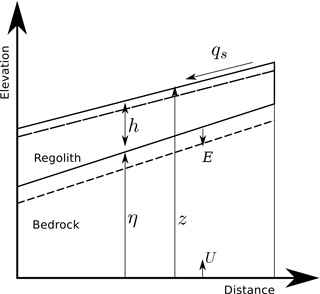Figure 1Diagram showing the conservation of mass within a 2-D domain where mass enters the system through uplift, U (units of m s−1), and exists as sediment transported, qs (m2 s−1), out of the domain. E (m s−1) is the rate of production of regolith, h (m) is the thickness of regolith, η (m) is the bedrock elevation, and z (m) is the total elevation.

2 Methods

## 2.1 Erosion within a single dimension system

We aim to understand the effects of the most basic assumptions of mass transport in landscape evolution on the sediment flux record. In other words, how do the response times vary for the advective stream power law and the diffusive transport model? To this end we derive the two models from first principles to demonstrate clearly how, from the same starting point, the fundamental assumptions made about mass transport initially give rise to very different model equations. We use this framework as a context for our investigation of an eroding system responding to precipitation change. We first define a one-dimensional system from which the basic equations can be assembled. Following we define a landscape of elevation z composed of bedrock, thickness η (units of metres), and a surface layer of sediment with thickness h (units of metres; see Fig. 1). This landscape is forced externally through uplift rate U (units of m yr−1). The bedrock is transferred into sediment through erosion at a rate E (units of m yr−1) and the sediment is transported across the system with a flux qs (units of m2 yr−1). Assuming that the densities of sediment and bedrock are equal, then the change in bedrock thickness is

$\begin{array}{}\text{(1)}& {\partial }_{\mathrm{t}}\mathit{\eta }=U-E,\end{array}$

and the rate of change in sediment thickness is

$\begin{array}{}\text{(2)}& {\partial }_{\mathrm{t}}h=E-{\partial }_{x}{q}_{\mathrm{s}}.\end{array}$

It then follows that the rate of change in landscape elevation is

$\begin{array}{}\text{(3)}& {\partial }_{\mathrm{t}}z={\partial }_{\mathrm{t}}\mathit{\eta }+{\partial }_{\mathrm{t}}h.\end{array}$

It is important to realize that to solve Eq. (3), we are required to make some assumptions that fundamentally affect the erosional dynamics of the modelled system, and we illustrate this below.

One basic assumption to make is that there is always a supply of transportable sediment; we can then follow through with the summation in Eq. (3), giving

$\begin{array}{}\text{(4)}& {\partial }_{\mathrm{t}}z=U-{\partial }_{x}{q}_{\mathrm{s}}.\end{array}$

This may be appropriate when modelling the transport of sediment along the riverbed and when considering the formation of alluvial fans (Guerit et al.2014; Paola et al.1992; Whipple and Tucker2002). In the absence of surface water we can assume that sediment flux is simply a function of local slope ${q}_{\mathrm{s}}=-\mathit{\kappa }{\partial }_{x}z$, where κ is the hill slope diffusion coefficient. In the presence of flowing water, the sediment flux is a function of the flowing water and local slope ${q}_{\mathrm{s}}=-c{q}_{\mathrm{w}}^{\mathit{\delta }}{\left({\partial }_{x}z\right)}^{\mathit{\gamma }}$, where c is the transport coefficient (units (m2 yr−1)1−n), qw is the water flux per unit width (units m2 yr−1), and the exponents δ>1 and γ≥1 are dependent on how sediment grains are transported along the bed . Furthermore, δ>1 is required to create concentrated flow . The change in landscape elevation is then given by

$\begin{array}{}\text{(5)}& {\partial }_{\mathrm{t}}z=U+{\partial }_{x}\left(\mathit{\kappa }{\partial }_{x}z+c{q}_{\mathrm{w}}^{\mathit{\delta }}{\left({\partial }_{x}z\right)}^{\mathit{\gamma }}\right),\end{array}$

which can be written as

$\begin{array}{}\text{(6)}& {\partial }_{\mathrm{t}}z=U+{\partial }_{x}\left(\left[\mathit{\kappa }+c{q}_{\mathrm{w}}^{\mathit{\delta }}{\left({\partial }_{x}z\right)}^{\mathit{\gamma }-\mathrm{1}}\right]{\partial }_{x}z\right).\end{array}$

Equation (6) is non-linear in the case that γ≠1. In deriving this equation of elevation change due to sediment transport we have simply summed the two terms for sediment flux, the linear and potentially non-linear slope-dependent terms. This summation has been done as it is the simplest way to generate landscape profiles that have the desired convex and concave elements observed in natural landscapes .

To solve this equation in one dimension we assume that the water flux is a function of the precipitation transported down the river network. The water collected is taken from the upstream drainage area, a, which is related to the main stream length, l, by lah, where h is the exponent taken from the empirical Hack's law (Hack1957). The main stream length is related to the longitudinal length of the catchment by lxd, where $\mathrm{1}\le d\le \mathrm{1.1}$ . Therefore, we can write $x\propto {a}^{h/d}$, and the water flux is the precipitation rate, α (m yr−1), multiplied by the length of the drainage system,

$\begin{array}{}\text{(7)}& {q}_{\mathrm{w}}={k}_{\mathrm{w}}\mathit{\alpha }{x}^{p},\end{array}$

where kw is a constant of proportionality with units m1−p and $p=d/h$. Furthermore it is observed that river catchments are typically longer than they are wide, and so p<2 . Therefore given that $\mathrm{0.5} (Rigon et al.1996), then $\mathrm{1.4}, and the transport model (Eq. 6) becomes

$\begin{array}{}\text{(8)}& {\partial }_{\mathrm{t}}z=U+{\partial }_{x}\left(\left[\mathit{\kappa }+c{k}_{\mathrm{w}}{\mathit{\alpha }}^{\mathit{\delta }}{x}^{p\mathit{\delta }}{\left({\partial }_{x}z\right)}^{\mathit{\gamma }-\mathrm{1}}\right]{\partial }_{x}z\right).\end{array}$

For simplicity, we will also assume kw=1 and vary c when exploring the model behaviour.

However, returning to Eq. (3), it is clear that the transport model is not the only solution. If we assume that the rate of change in sediment thickness is zero over geological timescales, which is to say all sediment created is transported out of the model domain, then Eq. (3) becomes

$\begin{array}{}\text{(9)}& {\partial }_{\mathrm{t}}z=U-E.\end{array}$

This assumption has been made previously when studying small mountain catchments where the river may erode directly into the bedrock. However, recent numerical studies, such as , have expanded this model to cover continent-scale landscapes.

It is clearly plausible to suppose that erosion is primarily due to flowing water, so the assumption of geologically instantaneous transport may well be valid for mass that is transported as suspended load within the water column. Such an assumption is less clear for bedload transport. We can assume that the speed at which suspended loads travel down-system is a function of the height achieved within each hop, which is a function of the water depth, settling velocity, and flow velocity. For small grains <1 mm, the settling velocity is given by the force balance between the weight of the grain and the viscous drag given by the Stokes law (Dietrich1982). For a particle of diameter $\mathrm{1}×{\mathrm{10}}^{-\mathrm{4}}$ m and density 2800 kg m−3 the settling velocity is ∼0.01 m s−1. Therefore the distance travelled assuming a flow velocity of 1 km h−1 and an elevation of suspension of 1 m is roughly 3 km. Using a similar argument the travel distance of a sediment grain typical of the Bengal Fan is estimated to be ∼104 m . This suggests that rapid transport of sediment across a continent is possible.

The percentage of mass transported in suspension may also be quite significant. For a small Alpine braided river it was found that the majority of mass was transported as suspended load , and for the river systems draining the Tian Shan, China, 70 % of mass is transported as suspended and dissolved load . Therefore significant mass may be transported rapidly, geologically instantaneously, down-system, suggesting that the assumption that ${\partial }_{\mathrm{t}}h\sim \mathrm{0}$ may be valid in some circumstances.

Assuming surface flow is the primary driver of landscape erosion and that positive x is in the downstream direction, then erosion, E, as a function of the power of the flow to detach particles of rock per unit width can be written as

$\begin{array}{}\text{(10)}& E=-{k}_{\mathrm{b}}{\mathit{\rho }}_{\mathrm{w}}g{q}_{\mathrm{w}}^{m}{\left({\partial }_{x}z\right)}^{n},\end{array}$

where kb is a dimensional constant that parameterizes bedrock erodibility (; units (m2 yr−1)1−m yr kg−1), ρw is water density, g is gravity, and m and n are constants. The exponent m∼0.5, as it is a function of how the stream flow width is proportional to the water flux (Lacey1930; Leopold and Maddock1953; Whittaker et al.2007). The exponent n>0 acts upon the slope.

In two dimensions the change in elevation is then given by

$\begin{array}{}\text{(11)}& {\partial }_{\mathrm{t}}z=U+k{q}_{\mathrm{w}}^{m}{\left({\partial }_{x}z\right)}^{n},\end{array}$

where the constant k lumps together the other constants (units m−1 (m2 yr−1)1−m), and if n≠1 Eq. (11) becomes non-linear. Using a version of Eq. (11) to invert river profiles for uplift histories, it is argued by some authors that n is close to unity . However, certain river profiles may arguably be indicative of n>1 (Lague2014). Furthermore if n>1, Eq. (11) becomes non-linear and the model response to precipitation rate change will become a function of both uplift and precipitation rates (Whipple2001).

To solve Eq. (11) in 1-D, as before we will assume that qw=kwαxp where $\mathrm{1.4}. The stream power law for landscape erosion in 1-D is then

$\begin{array}{}\text{(12)}& {\partial }_{\mathrm{t}}z=U+{k}_{p}{\mathit{\alpha }}^{m}{x}^{mp}{\left({\partial }_{x}z\right)}^{n},\end{array}$

where kp=kkwρwg (units mp (m2 yr−1)1−m).

We have demonstrated two different fundamental equations for change in elevation in 2-D (Eqs. 6 and 11) and the equivalent 1-D forms (Eqs. 8 and 12). These two models of elevation change differ in that Eq. (11) is an advection equation and Eq. (6) is a diffusion equation. This means that the time evolution of Eq. (11) would be a migrating wave of erosion travelling either up or down the catchment . This wave could also potentially take the form of a shock wave, in which due to the change in gradient the lower reaches of the migrating wave could travel faster than the upper reaches, creating a breaking wave . The time evolution of Eq. (6) is very different because here the evolution is dominated by diffusive processes. The diffusion coefficient is a function of the down-system collection of water, which can lead to the concentration of flow and the creation of realistic morphologies . It is not, however, completely established how the transport model responds differently to changes in precipitation forcing in comparison to the stream power model.

## 2.2 Linear and non-linear solutions

If n=1 (Eqs. 11 and 12) and γ=1 (Eqs. 6 and 8) then the models are linear, and we can solve the equations both analytically and in 1-D and 2-D numerical schemes. For the stream power model we use an implicit finite-difference scheme and for the transport model we use an explicit finite-element scheme with linear elements . If n≠1 and if γ≠1 the equations become non-linear. In this case the numerical solutions can become unstable for simple explicit schemes and may suffer from too much numerical diffusion for implicit schemes, unless the size of the time step is limited by the appropriate Courant–Friedrichs–Lewy (CFL) condition . Given the short time steps required to obtain an accurate solution, we explore the non-linear solutions for erosion down a river-long profile in 1-D. We solve for the stream power model (Eq. 12) using an explicit total variation diminishing scheme with the appropriate CFL condition . For the transport model (Eq. 8) we use an explicit finite-element model with quadratic elements and the appropriate CFL condition to find a stable solution.

## 2.3 Generalizing to a two-dimensional system

To solve Eqs. (6) and (11) over a 2-D domain requires an algorithm to route surface flow down the landscape. In our case, to explore how a model landscape responds to a change in precipitation rate we will make the simplest assumption available: that water flows down the steepest slope. We then solve for Eq. (11) using the numerical model Fastscape , with a resolution of 1000 by 1000 nodes for a 100 by 100 km domain, giving a spatial resolution of 100 m. Erosion by sediment transport in 2-D is solved following the MATLAB model of , which is available from . We solve Eq. (6) on a triangular grid with a resolution of 316 by 316 nodes for a 100 by 100 km domain, giving a spatial resolution of the order of 300 m. We also explored how the models evolve for a domain that is 500 by 500 km in size. The time step used for both models is 10 kyr.

We will explore how an idealized landscape evolves under uniform uplift at a rate of 0.1 mm yr−1. The initial condition is of a flat surface with a small amount of noise added to create a roughness. The boundary conditions are fixed elevation at the left and right sides and no flow at the sides. To explore the response of the two models to a change in precipitation rate we start the model with an initial precipitation rate of α0=1 m yr−1. For the linear models we then increase or decrease the precipitation rate to a new value, α1, after 10 Myr of model run time. This is to be sure that the steady state has been reached before applying the perturbation. For the non-linear models (Sects. 3.3 and 3.4), the precipitation rate is changed after 5 Myr as in this case steady state was reached earlier. As the coefficients c and k have units that are related to the exponents δ and m in Eqs. (6) and (11), respectively (Armitage et al.2013; Whipple and Meade2006), when modelling increasing values of δ and m the coefficients are likewise increased.

The response time for the transport model scales by the effective diffusivity and can be given by

$\begin{array}{}\text{(13)}& {\mathit{\tau }}_{\mathrm{t}}=\frac{{L}^{\mathrm{2}}}{\mathit{\kappa }+c{q}_{\mathrm{w}}^{\mathit{\delta }}},\end{array}$

where L is the model length scale (in this case the length of the domain). For the stream power model the response time is a function of the velocity at which the kinematic wave travels up the catchment (Whipple2001; Whipple and Tucker1999). The response time is therefore given by the time it takes for this wave of incision to travel up the catchment length, lc,

$\begin{array}{}\text{(14)}& {\mathit{\tau }}_{\mathrm{sp}}=\frac{{l}_{\mathrm{c}}}{k{q}_{\mathrm{w}}^{m}}.\end{array}$

Therefore we expect the response time to be a function of the choice of both the constants c and k and the exponents δ and m within both models. The effect of varying the coefficients m and δ independently has been previously explored (Armitage et al.2013; Whipple and Meade2006), and we therefore will not do so in detail again here. Instead we aim to compare the two models and therefore search for the values of c, k, m, and δ that generate similar topography at steady state. This steady state is then perturbed by a change in precipitation rate.

## 2.4 Generating similar landscapes

It has been previously demonstrated that both end-member models can generate convex-up long profiles (Crosby et al.2007; Kirkby1971; Smith and Bretherton1972; Smith et al.2000; Whipple and Tucker2002). From solving both Eqs. (8) and (12), where γ=1 and n=1, we find that in the range $\mathrm{1}<\mathit{\delta }\le \mathrm{1.5}$ and $\mathrm{0.3}\le m\le \mathrm{0.7}$ the two end-member models are comparable (see Appendix A). Given the possible additional degree of freedom introduced if we also vary γ and n, it is clear that river-long profiles are not a unique identifier of erosional processes. However, in order to compare how the end-member models respond to a change in precipitation rate, it is preferable to perturb catchments of a similar morphology. We will subsequently explore how the models in their linear and non-linear forms respond to a change in precipitation rates within the Results section.

### 2.4.1 Erosion by sediment transport

Six models have been run without a change in precipitation to find the steady-state topography. The models explored are first a set of three with varying δ and constant c, i.e. δ=1.1, 1.3, and 1.5 with $c={\mathrm{10}}^{-\mathrm{4}}$ (m2 yr${}^{-\mathrm{1}}{\right)}^{\mathrm{1}-\mathit{\delta }}$ (Fig. 2a), and a set of three in which δ and c co-vary, i.e. δ=1.1 with $c={\mathrm{10}}^{-\mathrm{2}}$ (m2 yr${}^{-\mathrm{1}}{\right)}^{\mathrm{1}-\mathit{\delta }}$, δ=1.3 with $c={\mathrm{10}}^{-\mathrm{3}}$ (m2 yr${}^{-\mathrm{1}}{\right)}^{\mathrm{1}-\mathit{\delta }}$, and δ=1.5 with $c={\mathrm{10}}^{-\mathrm{4}}$ (m2 yr${}^{-\mathrm{1}}{\right)}^{\mathrm{1}-\mathit{\delta }}$ (Fig. 2b). These values are chosen because they generate response times within the range of observations from normal fault-bounded sedimentary systems that have responded to changes in slip rate .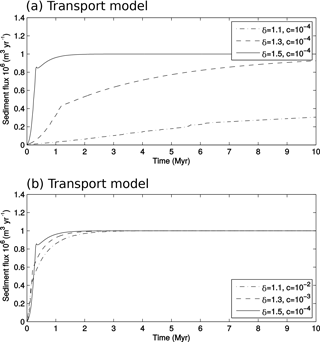Figure 2Sediment flux out of the model domain for the transport models in which (a) δ=1.1, 1.3, and 1.5, $\mathit{\kappa }={\mathrm{10}}^{-\mathrm{2}}$ and $c={\mathrm{10}}^{-\mathrm{4}}$ (m2 yr${}^{-\mathrm{1}}{\right)}^{\mathrm{1}-\mathit{\delta }}$; (b) δ=1.1, 1.3, and 1.5, $\mathit{\kappa }={\mathrm{10}}^{-\mathrm{2}}$ and $c={\mathrm{10}}^{-\mathrm{2}}$, 10−3, and 10−4 (m2 yr${}^{-\mathrm{1}}{\right)}^{\mathrm{1}-\mathit{\delta }}$.

When the transport coefficient c is the same for the three values of the exponent δ, the model wind-up time increases with decreasing δ and takes several million years when δ<1.5 (Fig. 2a). Steady-state sediment flux is greater for increasing δ when c is kept constant. The dimensions (units) of c depend on δ, which means that the value of the coefficient c must be adjusted when δ is changed to yield the same unit erosion rate per water flux regardless of δ (Armitage et al.2013). Consequently, when c is suitably adjusted the model can reach a steady state in a similar time for all three values of δ (Fig. 2b).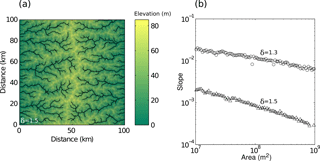Figure 3(a) Steady-state topography after 10 Myr for the transport model in which δ=1.5 and $c={\mathrm{10}}^{-\mathrm{4}}$ (m2 yr${}^{-\mathrm{1}}{\right)}^{\mathrm{1}-\mathit{\delta }}$. (b) Slope–area relationship for the transport model for δ=1.3 and δ=1.5.

We subsequently analyse the topography for the relationship between trunk river slope and drainage area, as shown in Fig. 3, using TopoToolbox2 . For the case in which δ=1.5 the scaling between channel slopes and catchment drainage areas, the slope–area exponent θ, is equal to 0.42, and for δ=1.3, θ is equal to 0.23 (Fig. 3b). The same value is calculated using the spatial transformation described in , commonly referred to as χ analysis (Table 1). Given the reduction in θ from δ=1.5 to 1.3, we did not analyse the case for δ=1.1 as the slope–area relationship will clearly lie below the observed range ($-\mathrm{0.7}<\mathit{\theta }<-\mathrm{0.35}$; e.g. ). Therefore, for river networks defined by routing water down the steepest slope of descent, the transport model can create catchment morphologies that have a concavity similar to that observed in nature if δ∼1.5.

Table 1Slope–area relationship for trunk streams derived using χ analysis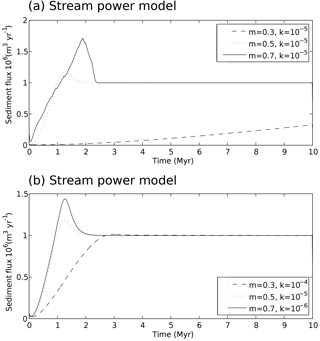Figure 4Sediment flux out of the model domain for the stream power models in which (a) m=0.3, 0.5, and 0.7 and $k={\mathrm{10}}^{-\mathrm{5}}$ (m2 yr${}^{-\mathrm{1}}{\right)}^{\mathrm{1}-m}$; (b) m=0.3, 0.5, and 0.7 and $k={\mathrm{10}}^{-\mathrm{4}}$, 10−5, and 10−6 m−1 (m2 yr${}^{-\mathrm{1}}{\right)}^{\mathrm{1}-m}$.

### 2.4.2 Comparison to erosion by stream power

In order to provide a comparison for the morphology of the transport model we explore how the stream power model evolves to a steady state. The landscape derived from the stream power model, as shown in Eq. (11), evolves towards a steady state with a slightly different behaviour in comparison to the transport model (Fig. 4). As before we ran six models for which in this case the first set of three are m=0.3, 0.5, and 0.7 with $k={\mathrm{10}}^{-\mathrm{5}}$ m−1 (m2 yr${}^{-\mathrm{1}}{\right)}^{\mathrm{1}-m}$ (Fig. 4a). The second set of three are of m=0.3 with $k={\mathrm{10}}^{-\mathrm{4}}$ m−1 (m2 yr${}^{-\mathrm{1}}{\right)}^{\mathrm{1}-m}$, m=0.5 with $k={\mathrm{10}}^{-\mathrm{5}}$ m−1 (m2 yr${}^{-\mathrm{1}}{\right)}^{\mathrm{1}-m}$, and m=0.7 with $k={\mathrm{10}}^{-\mathrm{6}}$ m−1 (m2 yr${}^{-\mathrm{1}}{\right)}^{\mathrm{1}-m}$ (Fig. 4b). This range of m is chosen as it spans the range of observed concavities within catchments. As with the transport model the coefficient k can be adjusted along with m as they are related, and increasing k reduces the model wind-up time (Fig. 4b). Decreasing the exponent m increases the timescale taken to reach a steady state (Fig. 4a); however, by varying k by a factor of 100, the steady-state sediment flux is reached within 3 Myr for the three values of m (Fig. 4b).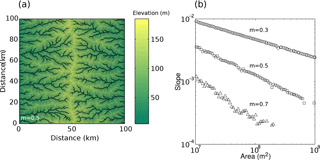Figure 5(a) Steady-state topography after 10 Myr for the transport model in which m=0.5 and $k={\mathrm{10}}^{-\mathrm{5}}$ m−1 (m2 yr${}^{-\mathrm{1}}{\right)}^{\mathrm{1}-m}$. (b) Slope–area relationship for the transport model for m=0.3, 0.5, and 0.7.

Following the previous examples, we analyse the topography for the relationship between trunk river slope and drainage area (Fig. 5). Both the transport model and the stream power model can create landscapes with similar slope–area relationships calculated using the χ-analysis approach (Table 1). For both models, the values of the intercept, ks, and the gradient, θ, are of similar magnitudes for δ=1.5 and m=0.5. Absolute elevation for the model shown in Fig. 5a is higher than the transport model example due to the larger value of k relative to c. However, importantly, both models can create similar landscape morphologies at steady state.

3 Results

The stream power and transport models can both fit the observed slope–area relationships of the present day landscape morphology, for example θ ranging from 0.35 to 0.7 , when the water flux exponent is m∼0.5 or δ∼1.5 for the stream power and transport model, respectively. Therefore, both models may be a reasonable representation of how, on a gross scale, a landscape erodes. We therefore keep the exponents in the range $\mathrm{0.3}\le m\le \mathrm{0.7}$ and $\mathrm{1.3}\le \mathit{\delta }\le \mathrm{1.5}$ and explore how the models in their linear and non-linear forms respond to a change in precipitation rates.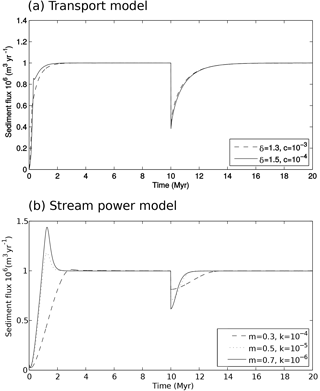Figure 6Response of the transport and stream power model to a reduction in precipitation rate. (a) Sediment flux for the transport model for a step reduction in precipitation from 1 to 0.5 m yr−1 after 10 Myr. Two models are plotted in which δ=1.3 and 1.5, $\mathit{\kappa }={\mathrm{10}}^{-\mathrm{2}}$, and $c={\mathrm{10}}^{-\mathrm{3}}$ and 10−4 (m2 yr${}^{-\mathrm{1}}{\right)}^{\mathrm{1}-\mathit{\delta }}$. (b) Sediment flux for the stream power model for a step reduction in precipitation from α0=1 to α1=0.5 m yr−1 after 10 Myr. Three models are plotted in which m=0.3, 0.5, and 0.7 and $k={\mathrm{10}}^{-\mathrm{4}}$, 10−5, and 10−6 m−1 (m2 yr${}^{-\mathrm{1}}{\right)}^{\mathrm{1}-m}$.

## 3.1 Response to precipitation rate reduction

When the model is perturbed by a change in precipitation rate the sediment flux output will first change as the erosive power changes (e.g. Fig. 6). The model will subsequently return to the steady-state output, as the slope of the fluvial system will adjust to the new precipitation rate, and the landscape will re-achieve the same steady state. In Fig. 6a we display the response of erosion for the transport model in terms of sediment flux out of the model domain for a reduction in precipitation rate from 1 to 0.5 m yr−1 at 10 Myr of model evolution. We explore how the transport model responds for δ=1.5, $c={\mathrm{10}}^{-\mathrm{4}}$ and δ=1.3, $c={\mathrm{10}}^{-\mathrm{3}}$ as these two values of δ generate reasonable slope–area relationships (Fig. 3b, Table 1). The response to a reduction in precipitation is similar for the two model parameter sets, with the flux initially reducing by half and then recovering to within 10 % of steady-state values within  2 Myr (Fig. 6a; see Table 2). Changing the transport coefficient, c, does not affect the predicted gradient of catchment slope versus catchment area (see Appendix A, Fig. A2). However, changing c changes the model elevation (Fig. A2). Furthermore, the larger the value of c the faster the response (Eq. 13; see ). A small increase in the exponent δ will strongly reduce response times, as it will increase the water flux term (Eq. 13). Therefore an order-of-magnitude decrease in c counters the change in δ for the two model sets (Fig. 6a). For the values chosen both models respond at a similar rate to the change in precipitation (Fig. 6a; see Table 2).

Table 2Response to a change in precipitation rate; α1 represents the value that the precipitation rate changes to from α0=1 mm yr−1. Response time is given for two model sizes, 100 and 500 km, and as the time for the model to recover to within 50 % (12) and 10 % (110) of the steady-state sediment flux.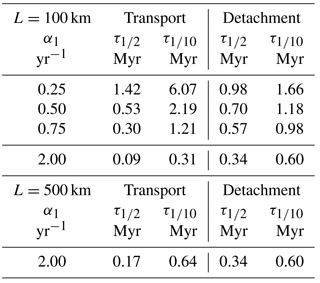The response of the stream power model to an identical reduction in precipitation at a model time of 10 Myr takes a similar form, with an initial decrease in sediment flux out followed by a gradual recovery (Fig. 6b). In a similar manner as the transport model, response is a function of the exponent m and the coefficient k (Eq. 14). We have modelled three parameter sets: m=0.3 and $k={\mathrm{10}}^{-\mathrm{4}}$, m=0.5 and $k={\mathrm{10}}^{-\mathrm{5}}$, and m=0.7 and $k={\mathrm{10}}^{-\mathrm{6}}$ (Fig. 6b). The response time to achieve a return to 10 % of the steady-state sediment flux varies from 3 Myr in the case of m=0.3 to less than 1 Myr when m=0.7. In addition to response time being longer for smaller values of m, the peak magnitude of the flux response is reduced for smaller values of m (Fig. 6b).

The magnitude of the response for all the runs is greater for the transport model when compared to the stream power model (Fig. 6). Consequently, response time, while being a function of the transport coefficients c and k, may still systematically differ between the two models: the transport model with δ=1.5 and $c={\mathrm{10}}^{-\mathrm{4}}$ generates a maximum model elevation of ∼240 m, and the stream power model with m=0.5 and $k={\mathrm{10}}^{-\mathrm{5}}$ generates a maximum elevation of ∼180 m. These two models have a similar slope–area relationship at steady state (Table 1) and are therefore comparable, suggesting a faster response to a reduction in precipitation rates for the stream power model (Fig. 6).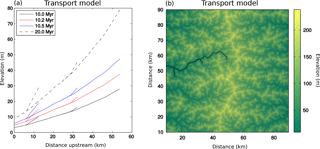Figure 7Transport model evolution due to a reduction in precipitation. (a) Selected river-long profile response to a change in precipitation. The black line is the profile just before a factor of 2 reduction in precipitation. The red and blue lines are 200 and 500 kyr after the reduction in precipitation. The dashed black line is the steady-state profile. (b) Trunk stream used for the analysis with the steady-state elevation.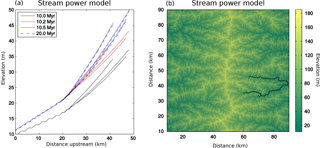Figure 8Stream power model evolution due to a reduction in precipitation. (a) Selected river-long profile response to a change in precipitation. The black line is the profile just before a factor of 2 reduction in precipitation. The red and blue lines are 200 and 500 kyr after the reduction in precipitation. The dashed black line is the steady-state profile. (b) Trunk stream used for the analysis with the steady-state elevation.

To explore how the difference in response time and magnitude is expressed in the landscape, we extract the river profiles of the main trunk systems for models in which δ=1.5 and m=0.5 during the response to the reduction in precipitation rate, while the uplift rate is constant (Figs. 7 and 8). For the transport model in which δ=1.5 and $c={\mathrm{10}}^{-\mathrm{4}}$, the catchment elevation increases to a new steady state that has an elevation that is roughly 2.6 times higher than the steady-state elevation after 10 Myr (Fig. 7). Just under half of this new topographic elevation is achieved within the first 500 kyr. In contrast, for the stream power model in which m=0.5 and $k={\mathrm{10}}^{-\mathrm{5}}$, the steady-state topography is achieved within a fraction of the time when compared to the transport model. This is in line with the more rapid response of this model to a relative drying of the climate using these parameters (compare Fig. 6a and b). Furthermore the increase in elevation due to the reduced surface water flux is only a factor of ∼1.2, which is less than half of the increase for the transport model. Our results confirm that two different end-member erosion models encompassing advective and diffusive phenomena can produce landscapes with similar morphologies if particular parameter sets are selected accordingly.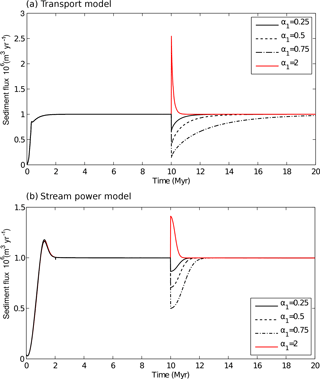Figure 9(a) Response of the transport model to a change in precipitation rate. Equation (6) is solved for δ=1.5 and $c=\mathrm{1}×{\mathrm{10}}^{-\mathrm{4}}$ (m2 yr${}^{-\mathrm{1}}{\right)}^{\mathrm{1}-\mathit{\delta }}$. The precipitation rate is initially α0=1 m yr−1 and changes to α1=0.25, 0.5, 0.75, or 2 m yr−1 after 10 Myr. (b) Response of the stream power model to a change in precipitation rate. Equation (6) is solved for m=0.5 and $k=\mathrm{1}×{\mathrm{10}}^{-\mathrm{5}}$ m−1 (m2 yr${}^{-\mathrm{1}}{\right)}^{\mathrm{1}-m}$. The precipitation rate is initially α0=1 m yr−1 and changes to α1=0.25, 0.5, 0.75, or 2 m yr−1 after 10 Myr.

## 3.2 Response to different magnitudes of precipitation rate change

The response time of the transport model is known to be a function of the transport coefficient and the magnitude of the precipitation rate (Armitage et al.2013). This behaviour is displayed in Fig. 9a, in which the response of the transport model with δ=1.5 and $c={\mathrm{10}}^{-\mathrm{4}}$ for a change in precipitation from 1 to 0.25, 0.5, 0.75, and 2 m yr−1 is plotted. The response time, measured as the time for the sediment flux to recover by half and by 90 % to the steady-state value, is shown additionally in Fig. 10 as black solid and dashed lines, respectively, and in Table 2. For a reduction to 0.25 m yr−1 the prediction is for a long response time of 6.07 Myr, while for an increase to 2 m yr−1 the prediction is a for rapid response time of 310 kyr for 90 % recovery towards previous sediment flux values. The equivalent half-life, recovery by 50 % towards previous sediment flux values, is 1.42 Myr and 90 kyr.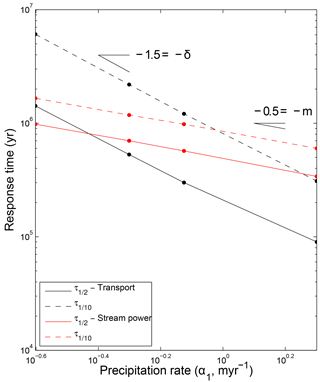Figure 10Log–log plot of response time to a change to a precipitation rate of α1 from an initial value of α0=1 m yr−1 when the model domain is 100 by 100 km (see Table 2); τ1∕2 is the time for the sediment flux to recover by half of the magnitude change in sediment flux and τ1∕10 is the time for the sediment flux to recover by 90 %.

The stream power model likewise has a response time that is a function of precipitation rate (Fig. 9b). For a reduction to 0.25 m yr−1 the prediction is for a response time of 1.66 Myr, while for an increase to 2 m yr−1 the prediction is for a recovery time of 600 kyr for 90 % recovery (Table 2). The equivalent half-life is 0.98 Myr and 340 kyr (Table 2). The stream power model is therefore faster to recover for a reduction in precipitation rate yet slower to respond to an increase in precipitation rate. This is because the response time of the stream power model is more weakly a function of precipitation rate. Importantly, these results therefore suggest that there is a fundamental asymmetry in the response timescale to a climate perturbation. The models suggest that it takes longer for surface processes to recover from a drying event compared to a wetting event.

Both models display a response time that is a function of the precipitation rate (Figs. 9 and 10). The relationship between precipitation rate and the transport model response can be expressed as

$\begin{array}{}\text{(15)}& {\mathit{\tau }}_{\mathrm{t}}\propto {\mathit{\alpha }}^{-\mathit{\delta }},\end{array}$

where in this case δ=1.5. This proportionality is in agreement with our numerical model results, in which the slope of trend for the transport model in the log–log plot is 1.5 (Fig. 10).

In contrast, the response time of the stream power model is not as strongly inversely dependent on the precipitation rate (Fig. 10). For this model, the response time is a function of the velocity at which the wave of incision travels upstream. This velocity is directly related to the inverse of the water flux, ${q}_{\mathrm{w}}^{m}$, which is in turn a function of the drainage length and precipitation rate, α. Therefore for the stream power model we can write that response time is

$\begin{array}{}\text{(16)}& {\mathit{\tau }}_{\mathrm{sp}}\propto {\mathit{\alpha }}^{-m}.\end{array}$

This proportionality, which is in agreement with the approximate analytical solutions of , is likewise in agreement with our numerical model results, in which the slope of trend for the stream power model in the log–log plot is 0.5 (Fig. 10). Consequently, for these two models, which were derived from the same starting point (Fig. 1) and applied to catchments of similar topography and morphology, we find that above a certain magnitude of precipitation rate change, the transport model responds more rapidly than the stream power model and vice versa.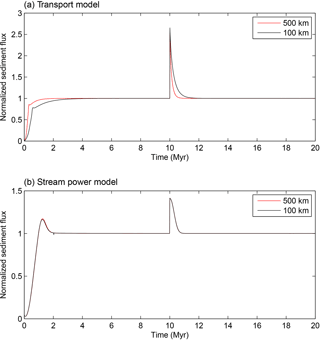Figure 11(a) Response of the transport model to a change in precipitation rate for two different model dimensions, 100 by 100 km and 500 by 500 km. Equation (6) is solved for δ=1.5 and $c=\mathrm{1}×{\mathrm{10}}^{-\mathrm{4}}$ (m2 yr${}^{-\mathrm{1}}{\right)}^{\mathrm{1}-\mathit{\delta }}$. The precipitation rate is initially α0=1 m yr−1 and changes to α1=2 m yr−1 after 10 Myr. (b) Response of the stream power model to a change in precipitation rate for two different model dimensions, 100 by 100 km and 500 by 500 km. Equation (6) is solved for m=0.5 and $k=\mathrm{1}×{\mathrm{10}}^{-\mathrm{5}}$ m−1 (m2 yr${}^{-\mathrm{1}}{\right)}^{\mathrm{1}-m}$. The precipitation rate is initially α0=1 m yr−1 and changes to α1=2 m yr−1 after 10 Myr.

The position of the critical point at which the stream power model responds more rapidly than the transport model is a function of the water flux and the collection of coefficients. In the model comparison developed here, we have compared two model catchments that have a similar slope–area exponent, θ between 0.4 and 0.5 (δ=1.5 and m=0.5) and model domain length of L=100 km, giving catchments of roughly 50 km length. In this case the 90 % recovery of the sediment flux signal is predicted to be more rapid for the transport model when compared to the stream power model for an increase in precipitation rate (Fig. 10). If, however, the model domain is increased to L=500 km then it takes twice as long for the transport model to recover from an increase in precipitation rate from 1 to 2 m yr−1: 0.63 Myr compared to 0.31 Myr for L=100 km (Fig. 11a and Table 2).

The stream power model is insensitive to the size of the model domain because of the particular choice of m=0.5 and the shape of drainage network that forms under the assumptions of routing water down the steepest slope of descent (Fig. 11b). Taking the drainage length to be directly proportional to the catchment area, lda, and given that catchment length is proportional to drainage area raised to the Hack exponent, h, we can re-write Eq. (14) as

$\begin{array}{}\text{(17)}& {\mathit{\tau }}_{\mathrm{sp}}\propto \frac{{a}^{h}}{{\left(\mathit{\alpha }a\right)}^{m}}.\end{array}$

Therefore, in the case that h=0.5 and m=0.5, as in the numerical model here, the response time becomes independent of system length (Whittaker and Boulton2012). If h<m then response times would decrease with increasing drainage basin size, and if h>m then response times would increase with drainage basin size. There is good empirical evidence for $\mathrm{0.5} (Rigon et al.1996), which fundamentally controls the plan view shape of catchments, yet there is not a complete consensus on the value of m (Lague2014; Temme et al.2017).

A final key difference between the transient sediment flux responses of the two models is that the peak magnitude of system response to a change in precipitation rate is systematically larger for the transport model (Fig. 9). For an increase in precipitation rates from 1 to 2 m yr−1, the sediment flux increases from 1×106 m3 to 2.5×106 m3 for erosion by sediment transport. This is 3 times greater than the equivalent increase for the stream power model. The reduction in sediment flux is likewise larger for the transport model (Fig. 9). Therefore, although response time is a function of precipitation rate, the magnitude of change is consistently larger for the transport model.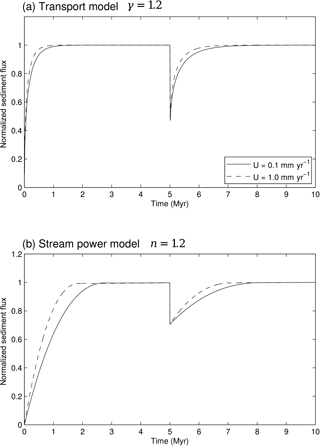Figure 12(a) Response of the transport model to a change in precipitation rate for two values of uplift, 0.1 and 1.0 mm yr−1. Equation (8) is solved for γ=1.2, δ=1.5, p=1.1, and $c=\mathrm{5}×{\mathrm{10}}^{-\mathrm{5}}$ (m2 yr${}^{-\mathrm{1}}{\right)}^{\mathrm{1}-\mathit{\delta }}$. The precipitation rate is initially α0=1 m yr−1 and changes to α1=0.5. (b) Response of the stream power model to a change in precipitation rate for two values of uplift, 0.1 and 1.0 mm yr−1. Equation (12) is solved for n=1.2, m=0.5, p=1.1, and $k=\mathrm{1}×{\mathrm{10}}^{-\mathrm{4}}$ m−1 (m2 yr${}^{-\mathrm{1}}{\right)}^{\mathrm{1}-m}$. The precipitation rate is initially α0=1 m yr−1 and changes to α1=0.5 after 5 Myr.

## 3.3 Non-linear response timescales

Up to this point we have compared how the models respond to a precipitation rate change when the solutions are linear. However, there is reasonable debate as to the value of the slope exponent n in the stream power model (Croissant and Braun2014; Lague2014; Rudge et al.2015) and likewise within the transport model it is plausible that the slope exponent γ>1. The response time for the stream power model for various values of n has been explored within . Here we expand on this by exploring the equivalent response times for the transport model. To explore the implications of the non-linearity introduced by relaxing the constraint that n=1 and γ=1 for both models, we solve Eqs. (8) and (12) for p=1.1, δ=1.5, and $c=\mathrm{5}×{\mathrm{10}}^{-\mathrm{5}}$ and m=0.5 and $k={\mathrm{10}}^{-\mathrm{4}}$, respectively, with different uplift rates. We have modelled the response due to an uplift rate between 0.1 and 1.0 mm yr−1 for the case in which γ=1.2 and n=1.2 in Eqs. (8) and (12) (Fig. 12).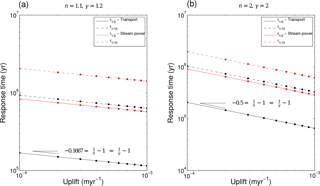Figure 13Log–log plot of response time for different slope exponents and uplift rates to a change to a precipitation rate from an initial value of α0=1 to α1=0.5 m yr−1; τ1∕2 is the time for the sediment flux to recover by half of the magnitude change in sediment flux and τ1∕10 is the time for the sediment flux to recover by 90 %. (a) Response time for the transport model (Eq. 8) and stream power model (Eq. 12) when the slope exponent γ=1.2 and n=1.2, respectively. A linear trend is found with a gradient of 1.667. (b) Response time for the transport model and stream power model when the slope exponent γ=2 and n=2, respectively. A linear trend is found with a gradient of 0.5.

We find that for both the transport and stream power model, when the slope exponent is greater than one, the model response time is a function of uplift rate. The faster the rate of uplift, the faster the system responds to a change in precipitation rate. If the response time for a system recovery to steady state by 50 or 10 % is plotted on a log–log plot against uplift rate we find that the response time is proportional to the uplift rate raised to a negative power (Fig. 13). In the case of n=1.2 or γ=1.2 the slope of trend is 0.1667, and for n=2 or γ=2 the slope of trend is 0.5 (Fig. 13). These slopes are in agreement with the approximate analytical solutions of and numerical models of ; i.e.  the stream power response time τsp has a proportionality,

$\begin{array}{}\text{(18)}& {\mathit{\tau }}_{\mathrm{sp}}\propto {U}^{\frac{\mathrm{1}}{n}-\mathrm{1}},\end{array}$

and equivalently we infer from our numerical model (Fig. 13) the transport model response time as

$\begin{array}{}\text{(19)}& {\mathit{\tau }}_{\mathrm{t}}\propto {U}^{\frac{\mathrm{1}}{\mathit{\gamma }}-\mathrm{1}}.\end{array}$

This implies that both models have the same form of response dependency on uplift rates. Therefore, regardless of the rate of uplift we should expect the transport model to respond more rapidly to a large increase in precipitation rate and the stream power model to respond more rapidly to a reduction in precipitation rate (Fig. 10). Our results are also consistent with the field-based findings of Whittaker and Boulton (2012), who showed that landscape response times for rivers close to the detachment-limited end-member were shorter for terrain uplifted by faster-slipping active faults.

## 3.4 Response time as a function of the initial precipitation rate

Up until this point we have only explored how the numerical models respond to an increase or decrease in precipitation rate by keeping the initial precipitation rate fixed at α0=1 m yr−1 and varying the final precipitation rate α1. In this final section we will instead keep the final precipitation rate fixed at α1=1 m yr−1 and vary the initial precipitation rate α0 from values of 0.5 to 1.5 m yr−1. We will focus again on the 1-D models and look at the linear and non-linear cases with n=1.2 and γ=1.2.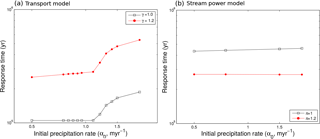Figure 14Log–log plots for the transport model and the stream power model in 1-D for a step change in precipitation rate; the initial precipitation rate, α0, varies from 0.5 to 1.5 m yr−1, and the final precipitation rate is fixed at α1=1 m yr−1. (a) Results for the transport model. (b) Results for the stream power model.

For the linear and non-linear transport model we find that if the initial precipitation is less than the final precipitation (α0<α1) then the response time is not very sensitive to the initial precipitation rate (Fig. 14a). If α0>α1 then the response time is a function of the initial precipitation rate, but the relationship cannot be explained by a simple power law (Fig. 14a). The change in response time as a function of the initial precipitation rate is, however, small compared to the change in response time as a function of the final precipitation rate.

In the case of the linear and non-linear stream power model, the response time has a no dependence on the initial precipitation rate and is only a function of the final precipitation rate (Fig. 14b). With all other parameters being held constant, the initial precipitation rate will set up the topography and hence the slope of the pre-perturbation landscape. Elevations will be lower for higher precipitation rates, and the topographic gradient will be smaller. For the case of the stream power model, the change in erosion rates migrates up the catchment and so the old topography does not impact the response time. For the transport model, however, the remnant topography does have a small effect on the response time, but only if the previous precipitation rate was higher than the new post-perturbation precipitation rate.

4 Discussion

In deriving the two end-member models to describe landscape evolution, we showed that if the rate of transport of sediment were assumed to be instantaneous (i.e. all sediment is transported out of the model domain) then the stream power model would be appropriate to describe catchment erosion. However, if it is instead assumed that the rate of sediment transport is not instantaneous, then we arrive at a model in which erosion scales with the rate of change of sediment flux, which itself is dependent on both linear and potentially non-linear slope-dependent terms. These two end-members can produce similar steady-state landscapes, as noted by a number of previous studies (Tucker and Whipple2002; Whipple and Tucker2002). However, as we demonstrate above, when perturbed by a change in conditions such as rainfall rate, they can produce very different landscape responses, which vary in terms of their style, magnitude, and tempo. We explore the nature and implications of these responses below.

It is also important to stress that the catchment responses and the predicted sediment fluxes out of these two model domains might be variously relevant to different erosional and depositional domains (Lague2014; Temme et al.2017). A model of instantaneous sediment transport might be more relevant for suspended sedimentary loads, for which transport times can be very small, while the transport model might be more appropriate for bedload-dominated systems, even in cases in which bedrock is clearly incised (Paola et al.1992; Valla et al.2010). Furthermore, given that these two models have different response times, it is possible that fine-grained deposits might record signals of climate change differently from, for example, the gravel deposits within alluvial valleys. Below, we will therefore first discuss how the two model responses compare in terms of their response time and place our results in the wider context of sediment routing system response to environmental change. Second, we will compare the model results with three records of change in sediment deposition during the Paleocene–Eocene thermal maximum (PETM), a known and well-constrained period of rapid climate change. Finally, we will summarize the key implications from our results.

## 4.1 Response times as a function of model choice

Under certain parameter sets it is relatively straightforward to generate two landscapes eroded by the transport or stream power model that have similar elevation, slope, and area metrics (Figs. 3 and 5). To find a path to break the apparent non-uniqueness of these solutions we have explored the transient sediment flux response out of the model domain for two end-member solutions to erosion. The first observation is that both models respond at a first order in a broadly similar way to a precipitation rate (climate) driver (Figs. 9 and 10). Both models have a response that is an inverse function of the magnitude of precipitation rate change. Both models have a response that is related to uplift in an identical manner (Fig. 13). However, the responses for catchments that are comparable in slope–area relationship and maximum elevation, but which are governed by different erosional dynamics defined by c, k, m, and δ, actually display different response times by almost 1 order of magnitude (Figs. 2 and 4).

We have demonstrated that models limited by their ability to transport sediment tend to have shorter response times to an increase in rainfall rate and thus re-achieve pre-perturbation sediment flux values more rapidly compared to stream-power-dominated systems, particularly when catchment length scales are small (e.g. <100 km, Fig. 10). These model observations suggest that the sediment fluxes from small alluvial catchments, even when captured in downstream depocentres, may be difficult to tie to changing climate parameters unless depositional chronologies are exceptionally well constrained (D'Arcy et al.2017). Conversely, catchments whose erosional dynamics lie close to the stream power end-member model may be well placed to record longer-term climate shifts, but may be buffered to very high-frequency variations in the climate driver (Armitage et al.2013; Simpson and Castelltort2012). It is important to stress that the trend in response is asymmetric, by which we mean that both models show a faster response for a precipitation increase relative to a precipitation decrease (Fig. 10). This is an important outcome, which has to date not been widely recognized or investigate in field scenarios. In particular, it raises the prospect that for glacial–interglacial cycles characterized by wetter, cooler stadial periods and dryer, warmer interstadials, the rapid climate recovery from peak glacial conditions typically seen in δ18O records might be mediated by a longer landscape response time to this change. Conversely, physically slower boundary condition changes towards wetter conditions may give rise to faster landscape response times. We suggest that an exploration of these differences may be a promising avenue of future research.

Given that the response time is a function of the water flux exponent (m or δ) and that the water flux exponent for the transport model is greater than that for the stream power model, there will be a cross-over point at which the stream power model responds faster than the transport model. This cross-over point is a function of the erodibility coefficient k and the transport coefficient c. In the scenario in which we have tried to initiate the perturbation in precipitation rates from similar catchments, we find that this cross-over point is towards large reductions in precipitation rates (Fig. 10). This implies that the transport model generally responds faster than the stream power model (105 to 106 yr) for examples in which the parameter combinations used here produce similar steady-state landscapes.

For such conditions, the stream power model predicts a landscape response time to a change in precipitation of the order of 106 yr, and this time is related to the precipitation rate to the inverse power of m (Fig. 10). The transport model predicts a wider range of response times of the order of 106 to 105 yr that is related to the precipitation rate to the inverse power of δ; in this case the response time is also length dependent (Fig. 10 and Table 2). It has been suggested that a transition from a landscape controlled by detachment-limited erosion (stream power model) to sediment transport at longer system lengths may explain the longevity of mountain ranges . This hypothesis is somewhat backed up by the analysis of response times for the transport model, as the response time increases with system length (Table 2) unlike the stream power model, which has a response that is only slightly modified by system length . To date, physical constraints on landscape and sediment flux response times to climate changes in the geologic past are relatively scarce because real systems are complex. They include internal dynamics, such as vegetation and autogenic behaviour, which are often omitted from model studies, and because of the need for stratigraphic archives to be complete with well-established chronologies . In principle, however, the dominant long-term incision process governing catchment behaviour fundamentally determines the sediment flux response and may itself help identify catchment erosional dynamics; we explore this question in Sect. 4.2.

Finally, it is worth noting that the model response time has implications for the inverse modelling of river profiles. When river-long profiles are inverted for uplift, erosion is typically assumed to be captured by the stream power model (Pritchard et al.2009). Studies of continent-scale inversion have found that the best fit value of k for the stream power model increases by 2 orders of magnitude to fit river profiles in Africa relative to Australia . Such a large change in k would result in a highly significant difference in response time from continent to continent, which in itself would imply that tectonic and climatic signals are preserved in landscapes and sediment archives over vastly different time periods (Demoulin et al.2017). Such an outcome may reflect fundamental differences in bedrock erodiblity , but alternatively could be satisfactorily explained by differing long-term erosional dynamics and sediment transport. These differences are enhanced in the case in which n>1 in the stream power erosion model.

## 4.2 Relevance of model responses to sediment records of climate change

To what extent do these model results, which start from similar steady-state topographies, help us to understand whether stratigraphic records of sediment accumulation through time do or do not reflect the effects of climatic change on sediment routing systems governed by differing long-term erosional dynamics? One motivation for this study has come from the increasing number of field and stratigraphic investigations of terrestrial sedimentary deposits, apparently contemporaneous with (and taken to record) known past climate perturbations, such as the Palaeocene–Eocene thermal maximum (PETM), a hyperthermal event that occurred around 56 Ma. Stratigraphers often correlate changing stratigraphic characteristics with changing environmental boundary conditions in a qualitative way (Allen2017; Romans et al.2016). However, to evaluate quantitatively how sediment routing systems respond to climate with reference to real examples, it is imperative to consider systems in which the timescales of erosion (or as a proxy, deposition) are known, stratigraphic sections are complete, and the driving mechanisms well documented (Allen et al.2013; D'Arcy et al.2017).

To compare our model predictions with observations it is clear that we have to use the depositional record. Therefore, there is an implicit assumption that stratigraphy is a faithful recorder of erosion. It is, however, possible that climatic change will also alter processes that control sediment deposition, for example by altering how sediment partitions from transport into stratigraphy. By using estimates of the total volume of sediment deposited within the Escanillia Eocene sedimentary system in the Spanish Pyrenees, it has been demonstrated that climatic change can recreate observed changes in grain size deposition . This example of a close model-to-stratigraphic-observation prediction might be evidence that the stratigraphic record is a faithful record of a change in sediment flux delivery to the depositional environment.

The PETM is arguably the most rapid global warming event of the Cenozoic, with a rise in global surface temperatures by 5 to 9 C , forming a clear step change in climate for which depositional records are well constrained in a number of basins worldwide . It is therefore a good example for high-level comparison with our model outputs. While the large-magnitude glacial–interglacial cycles of the past 1 Myr are also plausible candidates to investigate these links in principle (Armitage et al.2013), we note that many terrestrial records of sedimentation over ca. 100 kyr, such as fluvial terraces and alluvial fans, have depositional chronologies that are often incomplete or reworked .

The initial warming associated with the PETM event occurred at ca. 55.5 Ma and may have been as abrupt as 20 kyr, with a duration of 100 to 200 kyr based on the synthesis of δ13C and δ18O records (Foreman et al.2012; Schmitz and Pujalte2007). The event has been associated with clear changes to global weather patterns; for instance, hydrogen isotope records suggest increased moisture delivery towards the poles at the onset of the PETM, consistent with predictions of storm track migrations during global warming . This event has also been argued by an increasing number of authors to have produced a significant geomorphic and erosional impact based on sedimentary evidence and its apparent effect on the global hydrological cycle and catchment run-off (Foreman2014; Foreman et al.2012).

A clear response to the PETM is recorded within both the Spanish Pyrenees and the western US; however, the responses are arguably not the same. At the onset of the PETM there is strong evidence for the contemporaneous increase in precipitation rates and the deposition of coarse gravels known as the Claret Conglomerate in the Tremp Basin of the Spanish Pyrenees. In the western US the PETM is marked by the deposition of well-documented channel sandstone bodies in the Piceance Creek and Bighorn basins (Foreman2014; Foreman et al.2012). In the US cases, the deposits include coarse channelized sands, marked by upper flow regime bed forms, some of which are consistent with a synchronous increase in both water and sediment discharge. At Claret, where the style of sedimentation abruptly changes from a semi-arid alluvial plain to an extensive braid plain or megafan deposit, the conglomerate has a thickness of ∼10 m, while the total carbon isotope excursion (CIE) in the same section measures ∼35 m .

### 4.2.1 Claret Conglomerate, Spanish Pyrenees

The Claret Conglomerate was likely deposited rapidly, representing a fast response to climate change. If we assume a constant rate of deposition, then the Claret Conglomerate accounts for roughly 30 % of the total duration of deposition for the CIE (170 kyr; ), suggesting that deposition occurred over a duration of up to 50 kyr. Indeed, argue that the deposition of this unit may have been markedly quicker than the conservative estimate above, perhaps taking less than 10 kyr, based on their detailed comparison of δ13C and δ18O records at the field site compared to marine records of the excursion. Therefore unless there is a major unconformity within the CIE, the implication is that the erosional system responded rapidly at this particular field site, in 10 to 50 kyr, to a significant shift in climatic conditions. These values suggest sedimentation rates of up to 1 mm yr−1. If such a sedimentation rate had been sustained for the duration of the deposition of the Tremp Group (Maastrichtian to the end of the Palaeocene), the sediment thickness would be >15 km. This is an order of magnitude more than actually observed (Cuevas1992) and would therefore suggest that sediment fluxes increased dramatically at the PETM.

Erosional source catchment areas were likely <100 km in length given the palaeo-geography of the Pyrenees at the time . The very short duration of the erosional response, which is required for the sediments to be transported and deposited on a timescale of ca. 104 years, is therefore difficult to model within a stream power (advective) end-member model for catchments of this scale (Table 2), although a version of such a model has been recently used to explore the controls on the evolution of later Miocene megafans in the northern Pyrenees (Mouchené et al.2017). To use the stream power model would require a significant increase in the bedrock erodibility parameter, k, within the model (by greater than 1 order of magnitude), implying slopes and topography in the palaeo-Pyrenees that were highly subdued. In contrast, the sediment transport model more easily reproduces the documented response timescales given an increase in precipitation; it is also consistent with the volumetrically significant export of bedload-transported gravel clasts and therefore honours the independent field data more effectively. We also note that the transport model displays a response time that has a stronger dependence on precipitation rate change and has a greater amplitude of perturbation (e.g. Fig. 9). We therefore suggest that the erosional pulse that led to the deposition of the Claret Conglomerate is most appropriately modelled as a diffusive system response to a sharp increase in precipitation over the source catchments of the developing Pyrenean mountain chain at that time.

### 4.2.2 Sandstone bodies in the Piceance Creek and Bighorn basins, western US

The time-equivalent sections in the Bighorn and Piceance Creek basins of the western US also provide clear evidence of anomalous sedimentation at the PETM; however, in this case the duration of deposition is somewhat longer, >100 kyr . Here the deposits are of smaller grain sizes, with the boundary sandstone sequence in the Bighorn Basin being made up of fine to coarse sand with little gravel (Foreman2014). In the Piceance Creek basin, the PETM section documents the rapid progradation of coarse-grained sands, which is consistent with greater discharges, over silty underlying strata and in that sense these observations also match sediment transport model predictions for rapid increases in sediment flux driven by enhanced precipitation . However, it is notable that the documented changes in fluvial style persisted beyond the PETM and we therefore suggest that the fast response of the system to the increase in precipitation, but the persistence of coarser-grain sedimentation as the climate presumably dried and cooled, may indeed reflect the marked asymmetry in sediment flux responses to wetting and drying noted in Fig. 9.

In contrast, the Bighorn Basin boundary sandstone sediments are contained within the PETM time period and indicate uniform flow depths and widths during this time, while also being coarser than the underlying horizons. Moreover, proxy data suggest a net decrease rather than an increase in precipitation . While the progradation of such coarse-grained facies could be represented as a diffusive process driven by increasing rainfall-driven discharge , this is apparently inconsistent with the sedimentological characteristics of the deposit. Although sediment fluxes are not explicitly reconstructed in this work, this response apparently requires greater volumes and grain sizes of sediment delivered despite lowered rainfall conditions and is thus difficult to capture in either of the end-member models used here. Foreman et al. (2012) and Foreman (2014) argue for the preferential removal of fine-grained floodplain deposits speculatively linked to changing vegetation and the reduced cohesion of overbank sediments. Consequently, while two of the PETM sections considered here are broadly consistent with landscape responses governed by a sediment transport model, some depositional stratigraphies are not immediately consistent with either end-member model and may reflect important complexity, such as the effects of vegetation, lacking from simple model solutions.

## 4.3 Summary and model limitations

In this section we consider the implications of our model outputs, both generally for interpreting sediment routing system response to boundary condition change and specifically in the context of the well-studied PETM event. While the sediment flux response of the models to a change in precipitation are at a first-order level broadly similar, there are four key differences to highlight. First, starting from the same initial conditions, the sediment transport model appears to be more sensitive to precipitation change than the equivalent stream power model. It is therefore a good candidate for which rapid catchment-wide responses are recorded to, for example, a climate change event, as we argued for the PETM Claret Conglomerate in the Spanish Pyrenees. Second, we note that in both model cases there is a quicker response to a wetting than a drying event, something which has not been well established or demonstrated from field observations. Nevertheless we argue that field data sets, including PETM studies, may already have recorded this asymmetry, although it may not have been recognized as such. Third, the sediment transport model has a greater magnitude of peak sediment flux and is particularly sensitive to catchment size. Finally, we note that response time in both models is a function of uplift rate for n>1, which means that in such cases, perhaps counter-intuitively, the more perturbed the system the faster it responds (Whittaker and Boulton2012).

However, it is important to recognize that in deriving these two classic end-member models we have simplified landscape evolution considerably. We acknowledge that change in the model parameters, c, k, m, and δ, will alter the response times depicted here (Armitage et al.2013). However, in order to compare the two models we have specifically used values of c, k, m, and δ that generate comparable model landscapes, and we then changed the precipitation rate to understand the form of the model response. No model incorporates all the complexities that characterize sediment routing systems from source to sink (Allen2017) and the act of simplification inherent in considering erosional end-member models necessitates that in arguing for the applicability of one over the other, we simply consider the broad styles of behaviour suggested by model outputs. We do not, for example, consider autogenic behaviours (Forman and Straub2017), nor do we consider coupled issues of vegetation turnover in response to climate change, which may a play an important role in examples such as the Bighorn Basin considered here (Foreman2014). Nonetheless, a significant finding of this work has been the clear asymmetry in response time of these end-member models in terms of a wetting event (faster) compared to a drying event (slower). This implies that aridification events are harder to preserve in the sedimentary record, not only because they are typically associated with reduced sediment fluxes, but also because the timescale of landscape response may be >106 years.

5 Conclusions

Deterministic numerical models of landscape evolution rest on fundamental assumptions on how sediment is transported down-system. The stream power law is based on the assumption that all sediment generated is transported instantaneously out of the landscape. Transport models assume that there is an endless supply of sediment to be transported. The existence of knickpoints within river-long profiles, assumed to be produced by a system perturbation such as a base level, has been used to provide evidence in support of the stream power law in upland areas (Snyder et al.2000; Whipple and Tucker1999; Whittaker et al.2008). Knickpoints, however, can likewise be a result of changes in lithology and are certainly not a unique indicator of erosion dynamics (Grimaud et al.2016; Tucker and Whipple2002; Valla et al.2010). In this contribution we therefore attempted to understand how the sediment flux signal out of the eroding catchment may generate a distinguishable difference between the end-member models in terms of a response to a change in run-off. This idea is motivated from field observations of past landscape responses to climate excursions, such as the PETM, which are manifested in the rapid deposition of coarse sedimentary packages in terrestrial depocentres .

Both models suggest that the response time of landscape to a change in precipitation rate has a proportionality of the form of a negative power law (Eqs. 15 and 16). The key difference is in the value of the exponent. For the stream power model, the exponent must be less than one in order to match the observed concavity of river profiles. In contrast, for the transport model the exponent on the precipitation rate must be greater than one in order to generate a river network and to generate the observed concavity of river profiles. This results in the transport model responding more rapidly to an increase in precipitation rate in comparison to the stream power law model (Fig. 10). In contrast, the stream power model is faster to respond to a reduction in rainfall rate. This is fundamentally because the response time of this model is more weakly a function of precipitation than the sediment transport model. Significantly, therefore, our results show that there is a fundamental asymmetry in the response of both models to a climatic perturbation, with the response time to a drying event longer than that to an increase in rainfall. In general terms, the magnitude of the response to a change in precipitation rate appears greater across the range of model space investigated here for the sediment transport (diffusive) model solutions, while for the stream power (advective) model, the magnitude of the sediment flux perturbation is smaller, but is more localized within the catchment with respect to knickpoint retreat.

While this study does not address whether or not these sediment flux signals will be preserved in the stratigraphic record, a problem that fundamentally rests on the availability of accommodation to capture the eroded sediment (Allen et al.2013; Whittaker et al.2011), it does suggest that landscapes governed by these simple erosional end-members should be sensitive to climate change. Moreover, there are some important diagnostic differences between their sediment flux responses to an identical perturbation, including the amplitude, timescale, and locus of the erosional response. Using published stratigraphic examples, we suggest that the timescales and magnitude of coarse sediment deposition in the Spanish Pyrenees at the time of the PETM are best described using the diffusive transport model end-member. Moreover, we argue that these model end-members allow us to constrain the range of likely sediment flux scenarios that precipitation changes may generate and that numerical models, in conjunction with a range of field and independently constrained proxy data sets, are best placed to tease apart when and in what circumstances climate signals are likely to have been generated in erosional catchment systems, which fundamentally determines whether they can be subsequently captured in sedimentary depocentres downstream.

Code availability

The 1-D solution to the transport model is available from John Armitage (armitage@ipgp.fr). The 1-D solution to the stream power model is available from Benjamin Campforts (benjamin.compforts@kuleuven.be). Fastscape is available from Jean Braun (GFZ Potsdam) by request. The 2-D solution to the transport model was developed by Guy Simpson (University of Geneva) and is available as part of .

The solution to the one-dimensional stream power law (Eq. 12) assuming that at the end of the catchment at x=L elevation z=0 and mp≠1 is

$\begin{array}{}\text{(A1)}& {z}_{\mathrm{sss}}=\frac{U}{mk{\mathit{\alpha }}^{m}\left(mp-\mathrm{1}\right)}\left({x}^{\left(\mathrm{1}-mp\right)}-L{x}^{\left(\mathrm{1}-mp\right)}\right),\end{array}$

and for the case in which mp=1 this simplifies to

$\begin{array}{}\text{(A2)}& {z}_{\mathrm{sss}}=\frac{U}{k{\mathit{\alpha }}^{m}}{\mathrm{log}}_{e}\left(L/x\right).\end{array}$

For the transport model (Eq. 8) there is an exact solution for the case that δp=2, which assumes that at x=0, ${\partial }_{x}z=\mathrm{0}$ and at x=L, z=0:

$\begin{array}{}\text{(A3)}& {z}_{\mathrm{sst}}=-\frac{UL}{\mathrm{2}\mathit{\kappa }{D}_{\mathrm{e}}}\left(\mathrm{log}\left({D}_{\mathrm{e}}{x}^{\mathrm{2}}+\mathrm{1}\right)+\mathrm{log}\left({D}_{\mathrm{e}}+\mathrm{1}\right)\right),\end{array}$

where

$\begin{array}{}\text{(A4)}& {D}_{\mathrm{e}}=\frac{c{k}_{\mathrm{w}}{\mathit{\alpha }}^{\mathrm{2}/p}{L}^{\mathrm{2}}}{\mathit{\kappa }}.\end{array}$

For other values of δp the steady-state solution is solved numerically; Eq. (8) is solved using the finite-element method with linear weight functions. We use a non-uniform 1-D nodal spacing, for which the spatial resolution is increased with increasing gradient. The numerical model is bench-marked against the analytical solution for the case in which np=2.

The steady-state solutions are plotted in the case that $\mathit{\delta }=p=\sqrt{\mathrm{2}}$ and for reference the stream power model solution with m=0.5 and $p=\sqrt{\mathrm{2}}$ (Fig. A1). Such a value of p assumes that h∼0.7, which is towards the higher end for observed Hack exponents, and that the river catchment is very elongated. When plotting the logarithm of the model slope against drainage area (Fig. A1b), for which area is given by $a={x}^{p}/{k}_{\mathrm{w}}$ and assuming kw=1, for the simple stream power law derived here the slope–area exponent $\mathit{\theta }=-m$. The value of the dimensional constant k has no impact on the slope–area exponent as expected. The transport model likewise creates river-long profiles that have on average a negative curvature. For small values of x there is, however, a region of positive curvature in which κ>ckwαδLδp. For the slope–area analysis this leads to a positive gradient in the trend for small catchment areas. This relationship subsequently has a negative slope for larger catchments. The point of inflection is dependent on the value of De; for smaller values of κ the region of positive gradient is reduced. There is therefore a critical catchment area that is dependent on the diffusive term κ. After this critical point the slope–area relationship becomes negative. At distances down-system, where the upstream area is greater than this critical area, the gradient $\mathit{\theta }=-\mathrm{0.88}$; θ is insensitive to the coefficient c as would be expected.

The range of gradients found for river catchments for this type of slope–area analysis, usually referred to as concavity, generally lies within the range $\mathit{\theta }=-\mathrm{0.35}$ to 0.70 . It is trivial to find the values of m for the steady-state solution to the stream power law that fit such values of θ. To further explore how θ depends on δ and p within the transport model we solve Eq. (8) numerically for δ=1, 1.5, and 2 while keeping h=0.7 or 0.6 (Fig. A2). The result is that θ varies from 0.3 for the case of δ=1 to 1.31 for δ=2. The values of the gradient for the slope–area analysis for $\mathrm{1.4}, in which we assume d=1 and hence $p=\mathrm{1}/h$, are displayed in Table A1. For the transport model the slope is dependent on both δ and p.

Table A1Gradient, θ, of the slope vs. area trend at steady state for 1-D sediment transport (Eq. 8, Fig. A2).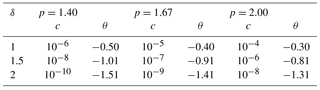Clearly there is a combination of δp values that is equally capable of fitting the observed river-long profile. Furthermore, for the transport model the slope is a function of the Hack exponent h (and therefore p) and the choice of δ. This because of the diffusivity term that leads to positive curvature and rounded 1-D profiles (Figs. A1b and A2). The magnitude of the water flux term within the transport equation (Eq. 8) is dependent on how much water the river network captures, which is in turn a function of how elongated the catchment is.

The positive slope–area relationship for the transport model for small catchment areas (see Figs. A1b and A2b) has been previously explored in . The gradient of the relationship between slope and catchment area is dominantly a function of the exponent δ within Eq. (6). The value of this exponent is likely within the range of $\mathrm{1}<\mathit{\delta }<\mathrm{2}$ depending on the bedload transport law assumed . If the observations of trunk river slope against catchment area are representative of a landscape at steady state, then for the smaller range of $\mathrm{1}<\mathit{\delta }\le \mathrm{1.5}$, a realistic catchment topography can be generated.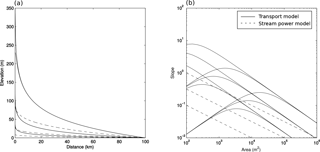Figure A1(a) Steady-state profiles of elevation against down-system length and (b) the slope of the profile plotted against the drainage area assuming that area a=xp, where $p=\mathrm{1}/h=\sqrt{\mathrm{2}}$ and h is the Hack exponent. Dashed lines are for the stream power law (Eq. 12) with m=0.5 and $k={\mathrm{10}}^{-\mathrm{4}}$, 10−3.5, and 10−3. The solid lines are for the transport model (Eq. 8 with $n=\sqrt{\mathrm{2}}$, $\mathit{\kappa }={\mathrm{10}}^{-\mathrm{3}}$, 1, and 103 m2 yr−1 and $c={\mathrm{10}}^{-\mathrm{6}}$, 10−5.5, and 10−5.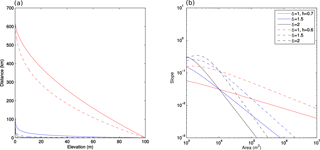Figure A2(a) Steady-state elevation and (b) slope–area relationship for the numerical solution to 1-D sediment transport (Eq. 8) for which the area, a, is taken to be related to distance x by a=xp, where $p=\mathrm{1}/h$ and h is the Hack exponent. Red lines are for the case in which n=1, blue lines for n=1.5, and black lines for n=2. Solid lines are for h=0.7. Dashed lines are for h=0.6.

Competing interests

The authors declare that they have no conflict of interest.

Acknowledgements

We would like to thank Guy Simpson (University of Geneva) for sharing his numerical model that solves the equations in two dimensions. We thank Tom Dunkley Jones (University of Birmingham) for discussions on the duration of conglomeratic deposition during the PETM, Gareth Roberts (Imperial College London) for discussions on slope exponents, and Jean Braun (GFZ Potsdam) for sharing his numerical model Fastscape. This work was initiated under funding by the Royal Astronomical Society through a research fellowship awarded to John Armitage while he was at Royal Holloway University of London.

Edited by: Jean Braun
Reviewed by: Laure Guerit and one anonymous referee

References

Allen, P. A.: Sediment Routing Systems: The Fate of Sediment from Source to Sink, Cambridge University Press, 2017. a, b

Allen, P. A., Armitage, J. J., Carter, A., Duller, R. A., Michael, N. A., Sinclair, H. D., Whitchurch, A. L., and Whittaker, A. C.: The Qs problem: Sediment volumetric balance of proximal foreland basin systems, Sedimentology, 60, 102–130, https://doi.org/10.1111/sed.12015, 2013. a, b, c, d

Armitage, J. J., Duller, R. A., Whittaker, A. C., and Allen, P. A.: Transformation of tectonic and climatic signals from source to sedimentary archive, Nat. Geosci., 4, 231–235, https://doi.org/10.1038/ngeo1087, 2011. a, b, c, d, e, f, g

Armitage, J. J., Dunkley Jones, T., Duller, R. A., Whittaker, A. C., and Allen, P. A.: Temporal buffering of climate-driven sediment flux cycles by transient catchment response, Earth Planet. Sci. Lett., 369–370, 200–210, https://doi.org/10.1016/j.epsl.2013.03.020, 2013. a, b, c, d, e, f, g, h, i, j, k

Armitage, J. J., Allen, P. A., Burgess, P. M., Hampson, G. J., Whittaker, A. C., Duller, R. A., and Michael, N. A.: Physical stratigraphic model for the Eocene Escanilla sediment routing system: Implications for the uniqueness of sequence stratigraphic architectures, J. Sediment. Res., 85, 1510–1524, https://doi.org/10.2110/jsr.2015.97, 2015. a, b

Baldwin, J. A., Whipple, K. X., and Tucker, G. E.: Implications of the shear stress river incision model for the timescale of postorogenic decay of topography, J. Geophys. Res., 108, 2158, https://doi.org/10.1029/2001JB000550, 2003. a, b, c, d, e

Banavar, J. R., Colaiori, F., Flammini, A., Giacometi, A., MAritan, A., and Rinaldo, A.: Sculpting of a fractal river basin, Phys. Rev. Lett., 23, 4522–4525, 1997. a

Bonnet, S. and Crave, A.: Landscape response to climate change: Insights from experimental modeling and implications for tectonic versus climatic uplift of topograph, Geology, 31, 123–126, 2003. a

Braun, J. and Willett, S. D.: A very efficient O(n), implicit and parallel method to solve the stream power equation governing fluvial incision and landscape evolution, Geomorphology, 180/181, 170–179, https://doi.org/10.1016/j.geomorph.2012.10.008, 2013. a, b

Braun, J., Voisin, C., Gourlan, A. T., and Chauvel, C.: Erosional response of an actively uplifting mountain belt to cyclic rainfall variations, Earth Surf. Dynam., 3, 1–14, https://doi.org/10.5194/esurf-3-1-2015, 2015. a

Campforts, B. and Covers, G.: Keeping the edge: A numerical method that avoids knickpoint smearing when solving the stream power law, J. Geophys. Res., 120, 1189–1205, https://doi.org/10.1002/2014JF003376, 2015. a, b

Castelltort, S. and Van Den Dreissche, J.: How plausible are high-frequency sediment supply-driven cycles in the stratigraphic record?, Sediment. Geol., 157, 3–13, https://doi.org/10.1016/S0037-0738(03)00066-6, 2003. a

Cowie, P. A., Whittaker, A. C., Attal, M., Roberts, G., Tucker, G. E., and Ganas, A.: New constraints on sediment-flux–dependent river incision: Implications for extracting tectonic signals from river profiles, Geology, 36, 535–538, https://doi.org/10.1130/G24681A.1, 2008. a

Croissant, T. and Braun, J.: Constraining the stream power law: a novel approach combining a landscape evolution model and an inversion method, Earth Surf. Dynam., 2, 155–166, https://doi.org/10.5194/esurf-2-155-2014, 2014. a

Crosby, B. T., Whipple, K. X., Gasparini, N. M., and Wobus, C. W.: Formation of fluvial hanging valleys: Theory and simulation, J. Geophys. Res., 112, F03510, https://doi.org/10.1029/2006JF000566, 2007. a

Cuevas, J. L.: Estratigrafia del “Garumniense” de la Conca de Tremp, Prepirineo de Lerida, Acata Geological Hispanica, 27, 95–108, 1992. a

D'Arcy, M., Whittaker, A. C., and Roda-Boluda, D. C.: Measuring alluvial fan sensitivity to past climate changes using a self-similarity approach to grain-size fining, Death Valley, California, Sedimentology, 64, 388–424, https://doi.org/10.1111/sed.12308, 2016. a

D'Arcy, M., Whittaker, A. C., and Roda-Boluda, D. C.: Measuring alluvial fan sensitivity to past climate changes using a self-similarity approach to grain-size fining, Death Valley, California, Sedimentology, 64, 388–424, https://doi.org/10.1111/sed.12308, 2017. a, b, c

Demoulin, A., Mather, A., and Whittaker, A.: Fluvial archives, a valuable record of vertical crustal deformation, Quaternary Sci. Rev., 166, 10–37, https://doi.org/10.1016/j.quascirev.2016.11.011, 2017. a, b, c

Densmore, A. L., Allen, P. A., and Simpson, G.: Development and response of a coupled catchment fan system under changing tectonic and climatic forcing, J. Geophys. Res., 112, F01002, https://doi.org/10.1029/2006JF000474, 2007. a

Dietrich, W. E., Bellugi, D. G., Sklar, L. S., Srock, J. D., Heimsath, A. M., and Roering, J. J.: Geomorphic transport laws for predicting landscape form and dynamics, in: Prediction in Geomorphology, edited by: Wilcock, P. R. and Iverson, R. M., Geoph. Monog. Series, 135, 1–30, https://doi.org/10.1029/135GM09, 2003. a

Dietrich, W. M.: Settling velocity of natural particles, Water Resour. Res., 18, 1615–1626, 1982. a

Dodds, P. S. and Rothman, D. H.: Geometry of river networks. I. Scaling, fluctuations and deviations, Phys. Rev. E, 63, 016115, https://doi.org/10.1103/PhysRevE.63.016115, 2000. a, b, c

Dunkley Jones, T., Ridgwell, A., Lunt, D. J., Maslin, M. A., Schmidt, D. N., and Valdes, P. J.: A Palaeogene perspective on climate sensitivity and methane hydrate instability, P. T. Roy. Soc. Pt. A, 368, 2395–2415, https://doi.org/10.1098/rsta.2010.0053, 2010. a

Foreman, B. Z.: Climate-driven generation of a fluvial sheet sand body at the Paleocene-Eocene boundary in north-west Wyoming (USA), Basin Res., 26, 225–241, https://doi.org/10.1111/bre.12027, 2014. a, b, c, d, e, f

Foreman, B. Z., Heller, P. L., and Clementz, M. T.: Fluvial response to abrupt global warming at the Palaeocene/Eocene boundary, Nature, 491, 92–95, https://doi.org/10.1038/nature11513, 2012. a, b, c, d, e, f, g, h, i

Forman, B. Z. and Straub, K. M.: Autogenic geomorphic processes determine the resolution and fidelity of terrestrial paleoclimate records, Sci. Adv., 3, e1700683, https://doi.org/10.1126/sciadv.1700683, 2017. a, b

Ganti, V., Lamb, M. P., and McElroy, B.: Quantitative bounds on morphodynamics and implications for reading the sedimentary record, Nat. Comun., 5, 3298, https://doi.org/10.1038/ncomms4298, 2014. a, b

Grimaud, J. L., Chardon, D., and Beauvais, A.: Very long-term incision of big rivers, Earth Planet. Sci. Lett., 405, 74–84, https://doi.org/10.1016/j.epsl.2014.08.021, 2014. a

Grimaud, J. L., Paola, C., and Voller, V.: Experimental migration of knickpoints: influence of style of base-level fall and bed lithology, Earth Surf. Dynam., 4, 11–23, https://doi.org/10.5194/esurf-4-11-2016, 2016. a

Guerit, L., Métivier, F., Devauchelle, O., Lajeunesse, E., and Barrier, L.: Laboratory alluvial fans in one dimension, Phys. Rev. E, 90, 022203, https://doi.org/10.1103/PhysRevE.90.022203, 2014. a

Hack, J. T.: Studies of longitudingal profiles in Virginia and Maryland, US Geological Survey Proffesional Papaer, 294-B, 1957. a

Hancock, G. R., Coulthard, T. J., and Lowry, J. B. C.: Predicting uncertainty in sediment transport and landscape evolution - the influence of initial surface conditions, Comput. Geosci., 90, 117–130, https://doi.org/10.1016/j.cageo.2015.08.014, 2016. a

Howard, A. D. and Kerby, G.: Channel changes in badlands, Geol. Soc. Am. Bull., 94, 739–752, 1983. a, b

Izumi, N. and Parker, G.: Inception of channelization and drainage basin formation: upstream-driven theory, J. Fluid Mech., 283, 341–363, 1995. a

Kirkby, M. J.: Hillslope process-response models based on the continuity equation, Special Publication Institute of British Geographers, 3, 15–30, 1971. a

Lacey, G.: Stable channels in alluvium, in: Minutes of the Proceedings, edited by: Grierson, W. W., Institution of Civil Engineers Publishing, vol. 229, 259–292, 1930. a

Lague, D.: The stream power river incision model: evidence, theory and beyond, Earth Surf. Proc. Land., 39, 38–61, https://doi.org/10.1002/esp.3462, 2014. a, b, c, d

Leopold, L. B. and Maddock, T.: The hydraulic geometry of stream channels and some physiographic implications, Tech. Rep. 252, US Geological Survey Proffesional Papers, 57 pp., 1953. a

Liu, Y., Métivier, F., Gaillerdet, J., Ye, B., Meunier, P., Narteau, C., Lajeunesse, E., Han, T., and Malverti, L.: Erosion rates deduced from seasonal mass balance along the upper Urumqi River in Tianshan, Solid Earth, 2, 283–301, https://doi.org/10.5194/se-2-283-2011, 2011. a

Manners, H. R., Grimes, S. T., Sutton, P. A., Domingo, L., Leng, M. J., Twitchett, R. J., Hart, M. B., Dunkley Jones, T., Pancost, R. D., Duller, R., and Lopez-Martinez, N.: Magnitude and profile of organic carbon isotope records from the Paleocene – Eocene Thermal Maximum: Evidence from northern Spain, Earth Planet. Sci. Lett., 376, 220–230, https://doi.org/10.1016/j.epsl.2013.06.016, 2013. a, b

Maritan, A., Rinaldo, A., Rigon, R., Giacometti, A., and Rordriguez-Iturbe, I.: Scaling laws for river networks, Phys. Rev. E, 53, 1510–1515, 1996. a

Meunier, P., Métivier, F., Lajeunesse, E., Mériaux, A. S., and Faure, J.: Flow pattern and sediment transport in a braided river: The ”torrent de St Pierre” (French Alps), J. Hydrol., 330, 496–505, https://doi.org/10.1016/j.jhydrol.2006.04.009, 2006. a

Michael, N. A., Whittaker, A. C., Carter, A., and Allen, P. A.: Volumetric budget and grain-size fractionation of a geological sediment routing system: Eocene Escanilla Formation, south-central Pyrenees, Geol. Soc. Am. Bull., 126, 585–599, https://doi.org/10.1130/B30954.1, 2014. a

Mouchené, M., van der Beek, P., Carretier, S., and Mouthereau, F.: Autogenic versus allogenic controls on the evolution of a coupled fluvial megafan-mountainous catchment system: numerical modelling and comparison with the Lannemezan megafan system (northern Pyrenees, France), Earth Surf. Dynam., 5, 125–143, https://doi.org/10.5194/esurf-5-125-2017, 2017. a

Paola, C., Heller, P. L., and Angevine, C. L.: The large-scale dynamics of grain-size variation in alluvial basins, 1: Theory, Basin Res., 4, 73–90, https://doi.org/10.1111/j.1365-2117.1992.tb00145.x, 1992. a, b, c, d, e

Pastor-Satorras, R. and Rothman, D. H.: Stochastic Equation for the Erosion of Inclined Topography, Phys. Rev. Lett., 80, 4349–4352, https://doi.org/10.1103/PhysRevLett.80.4349, 1998. a

Perron, J. T. and Royden, L.: An integral approach to bedrock river profile analysis, Earth Surf. Proc. Land., 38, 570–576, https://doi.org/10.1002/esp.3302, 2012. a, b

Pritchard, D., Roberts, G. G., White, N. J., and Richardson, C. N.: Uplift histories from river profiles, Geophys. Res. Lett., 36, L24301, https://doi.org/10.1029/2009GL040928, 2009. a, b

Rigon, R., Rodriguez-Iturbe, I., Maritan, A., Giacometti, A., Tarboton, D. G., and Rinaldo, A.: On Hack's law, Water Resour. Res., 32, 3367–3374, 1996. a, b

Rohais, S., Bonnet, S., and Eschard, R.: Sedimentary record of tectonic and climatic erosional perturbations in an experimental coupled catchment-fan system, Basin Res., 23, 1–15, https://doi.org/10.1111/j.1365-2117.2011.00520.x, 2011. a

Röhl, U., Westerhold, T., Bralower, T. J., and Zachos, J. C.: On the duration of the Paleocene-Eocene thermal maximum (PETM), Geochem. Geophy. Geosy., 8, Q12002, https://doi.org/10.1029/2007GC001784, 2007. a

Romans, B. W., Castelltort, S., Covault, J. A., Fildani, A., and Walsh, J. P.: Environmental signal propagation in sedimentary systems across timescales, Earth-Sci. Rev., 153, 7–29, https://doi.org/10.1016/j.earscirev.2015.07.012, 2016. a, b, c

Roy, S. G., Koons, P. O., Upton, P., and Tucker, G. E.: The influence of crustal strength fields on the patterns and rates of fluvial incision, J. Geophys. Res., 120, 275–299, https://doi.org/10.1002/2014JF003281, 2015. a, b

Rudge, J. F., Roberts, G. G., White, N. J., and Richardson, C. N.: Uplift histories of Africa and Australia from linear inverse modeling of drainage inventories, J. Geophys. Res., 120, 894–914, https://doi.org/10.1002/2014JF003297, 2015. a, b, c, d

Schmitz, B. and Pujalte, V.: Abrupt increase in seasonal extreme precipitation at the Paleocene-Eocene boundary, Geology, 35, 215–218, https://doi.org/10.1130/G23261A.1, 2007. a, b, c

Schwanghart, W. and Scherler, D.: Short Communication: TopoToolbox 2 – MATLAB-based software for topographic analysis and modeling in Earth surface sciences, Earth Surf. Dynam., 2, 1–7, https://doi.org/10.5194/esurf-2-1-2014, 2014. a

Simpson, G.: Practical Finite Element Modeling in Earth Science using Matlab, Wiley-Blackwell, 2017. a, b

Simpson, G. and Castelltort, S.: Model shows that rivers transmit high-frequency climate cycles to the sedimentary record, Geology, 40, 1131–1134, https://doi.org/10.1130/G33451.1, 2012. a, b

Simpson, G. and Schlunegger, F.: Topographic evolution and morphology of surfaces evolving in response to coupled fluvial and hillslope sediment transport, J. Geophys. Res., 108, 2300, https://doi.org/10.1029/2002JB002162, 2003. a, b

Sluijs, A., Schouten, S., Pagani, M., Woltering, M., Brinkhuis, H., Sinninghe Damsté, J. S., Dickens, G. R., Huber, M., Reichart, G. J., Stein, R., Matthiessen, J., Lourens, L. J., Pedentchouk, N., Backman, J., Moran, K., and the Expedition 302 scientists: Subtropical Arctic Ocean temperatures during the Palaeocene/Eocene thermal maximum, Nature, 411, 610–613, https://doi.org/10.1038/nature04668, 2006. a

Smith, T. R.: A theory for the emergence of channelized drainage, J. Geophys. Res., 115, F02023, https://doi.org/10.1029/2008JF001114, 2010. a

Smith, T. R. and Bretherton, F. P.: Stability and conservation of mass in drainage basin evolution, Water Resour. Res., 8, 1506–1529, https://doi.org/10.1029/WR008i006p01506, 1972. a, b, c, d, e, f, g, h, i

Smith, T. R., Merchant, G. E., and Birnir, B.: Transient attractors: towards a theory of the graded stream for alluvial and bedrock channels, Comput. Geosci., 26, 541–580, https://doi.org/10.1016/S0098-3004(99)00128-4, 2000. a, b

Snyder, N. P., Whipple, K. X., Tucker, E., and Merrits, D. J.: Landscape response to tectonic forcing: Digital elevation model analysis of stream profiles in the Mendocino triple junction region, northern California, Geol. Soc. Am. Bull., 112, 1250–1263, https://doi.org/10.1130/0016-7606(2000)112<1250:LRTTFD>2.0.CO;2, 2000. a, b, c, d

Tarboton, D. G., Bras, R. L., and Rodriguez-Iturbe, I.: Comment on “On the fractal dimension of stream network” by Paolo La Barbera and Renzo Rosso, Water Resour. Res., 26, 2243–2244, https://doi.org/10.1029/WR026i009p02243, 1990. a

Temme, A. J. A. M., Armitage, J. J., Attal, M., van Gorp, W., Coulthard, T. J., and Schoorl, J. M.: Choosing and using landscape evolution models to inform field stratigraphy and landscape reconstruction studies, Earth Surf. Proc. Land., 42, 2167–2183, https://doi.org/10.1002/esp.4162, 2017. a, b, c

Tinkler, K. J. and Whol, E. E.: Rivers over Rock: fluvial processes in bedrock channels, American Geophysical Union, Washington, USA, Geophys. Monog. Series, 107, https://doi.org/10.1029/GM107, 1998. a

Tucker, G. E. and Whipple, K. X.: Topographic outcomes predicted by stream erosion models: Sensitivity analysis and intermodel comparison, J. Geophys. Res., 107, 2179, https://doi.org/10.1029/2001JB000162, 2002.  a, b, c, d

Valla, P. G., van der Beek, P. A., and Lague, D.: Fluvial incision into bedrock: Insights from morphometric analysis and numerical modeling of gorges incising glacial hanging valleys (Western Alps, France), J. Geophys. Res., 116, F02010, https://doi.org/10.1029/2008JF001079, 2010. a, b

van der Beek, P. and Bishop, P.: Ceonzoic river profile development in the Upper Lachlan catchment (SE Australia) as a test of quantative fluvial incision models, J. Geophys. Res., 108, 2309, https://doi.org/10.1029/2002JB002125, 2003. a

Whipple, K. X.: Fluvial landscape response time: how plausible is steady-state denudation, Am. J. Sci., 301, 313–325, 2001. a, b, c, d, e, f

Whipple, K. X.: Bedrock rivers and the geomorphology of active orogens, Ann. Rev. Earth Planet. Sci., 32, 151–185, https://doi.org/10.1146/annurev.earth.32.101802.120356, 2004. a

Whipple, K. X. and Meade, B. J.: Orogen response to changes in climatic and tectonic forcing, Earth Planet. Sci. Lett., 243, 218–228, https://doi.org/10.1016/j.epsl.2005.12.022, 2006. a, b

Whipple, K. X. and Tucker, G. E.: Dynamics of the stream-power river incision model: Implications for height limits of mountain ranges, landscape response times, and research needs, J. Geophys. Res., 104, 17661–17674, 1999. a, b

Whipple, K. X. and Tucker, G. E.: Implications of sediment-flux-dependent river incision models for landscape evolution, J. Geophys. Res., 107, https://doi.org/10.1029/2000JB000044, 2002. a, b, c, d, e

Whittaker, A. C. and Boulton, S. J.: Tectonic and climatic controls on knickpoint retreat rates and landscape response times, J. Geophys. Res., 117, F02024, https://doi.org/10.1029/2011JF002157, 2012. a, b, c

Whittaker, A. C., Cowie, P. A., Attal, M., Tucker, G. E., and Roberts, G. P.: Bedrock channel adjustment to tectonic forcing: Implications for predicting river incision rates, Geology, 35, 103–106, https://doi.org/10.1130/G23106A.1, 2007. a, b

Whittaker, A. C., Attal, M., Cowie, P. A., Tucker, G. E., and Roberts, G.: Decoding temporal and spatial patterns of fault uplift using transient river long-profiles, Geomorphology, 100, 506–526, https://doi.org/10.1016/j.geomorph.2008.01.018, 2008. a

Whittaker, A. C., Duller, R. A., Springett, J., Smithells, R. A., Whitchurch, A. L., and Allen, P. A.: Decoding downstream trends in stratigraphic grain size as a function of tectonic subsidence and sediment supply, Geol. Soc. Am. Bull., 123, 1363–1382, 2011. a, b

Willett, S. D., McCoy, S. W., Perron, J. T., Goren, L., and Chen, C.: Dynamic Reorganization of River Basins, Science, 343, 6175, https://doi.org/10.1126/science.1248765, 2014. a

Willgoose, G., Bras, R. L., and Rodriguez-Iturbe, I.: A physical explanation of an observed link area-slope relationship, Water Resour. Res., 27, 1697–1702, 1991. a

Wobus, C., Whipple, K. X., Kirby, E., Snyder, N., Johnson, J., Spyropolou, K., Crosby, B., and Sheehan, D.: Tectonics from topography: Procedures, promise, and pitfalls, Geol. Soc. Am. Sp., 398, 55–74, 2006. a, b, c